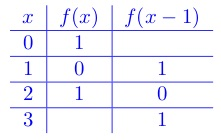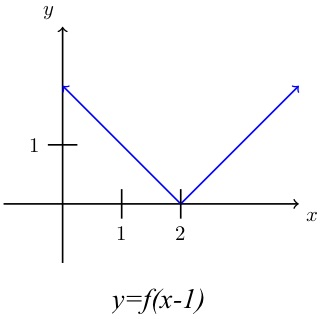# Horizontal Transformation: Sliding a Function Sideways

## Question

This is the graph ofSketch the graph of .

## Solution

The change from  to  is called a horizontal transformation; it slides the function sideways. The graph of  lies one unit to the right of the graph of . This may be counterintuitive, so let’s look at some numbers. At each x-value, the graph of  takes the y-value that the graph of  takes one unit earlier. Some values on the two graphs look like this:The y-values from the original graph have all chugged along to correspond to higher x-values than they did before.At any x-value on the graph, the function  operates on the  value 1 unit to the left of that x-value. That has the effect of moving the curve 1 unit to the right.

Similarly,  graphs one unit to the left of . Note that this is different from  which graphs one unit up from  because the 1 that gets added to the function means 1 gets added to every y-value. Similarly,  graphs one unit below  If you don’t believe me on any of these, try playing around with values of a simple function to see what happens.